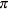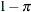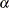MODEL Statement

Subsections:

MODEL response <(response-options)> = <effects> </ model-options> ;

MODEL events/trials = <effects> </ model-options> ;

MODEL + <effects> </ model-options> ;

The MODEL statement defines elements of the mixture model, such as the model effects, the distribution, and the link function. At least one MODEL statement is required. You can specify more than one MODEL statement. Each MODEL statement identifies one or more components of a mixture. For example, if components differ in their distributions, link functions, or regressor variables, then you can use separate MODEL statements to define the components. If the finite mixture model is homogeneous—in the sense that all components share the same regressors, distribution, and link function—then you can specify the mixture model with a single MODEL statement by using the K= option.

An intercept is included in each model by default. It can be removed with the NOINT option.

The dependent variable can be specified by using either the response syntax or the events/trials syntax. The events/trials syntax is specific to models for binomial-type data. A binomial(n,) variable is the sum of n independent Bernoulli trials with event probability. Each Bernoulli trial results in either an event or a nonevent (with probability). The value of the second variable, trials, gives the number n of Bernoulli trials. The value of the first variable, events, is the number of events out of n. The values of both events and (trialsevents) must be nonnegative, and the value of trials must be positive. Other distributions that allow the events/trials syntax are the beta-binomial distribution and the binomial cluster model.

If the events/trials syntax is used, the FMM procedure defaults to the binomial distribution. If you use the response syntax, the procedure defaults to the normal distribution unless the response variable is a character variable or listed in the CLASS statement.

The FMM procedure supports a continuation-style syntax in MODEL statements. Because a mixture has only one response variable, it is sufficient to specify the response variable in one MODEL statement. Other MODEL statements can use the continuation symbol + before the specification of effects. For example, the following statements fit a three-component binomial mixture model:

class A;
model y/n = x / k=2;
model     + A;


The first MODEL statement uses the = sign to separate response from effect information and specifies the response variable by using the events/trials syntax. This determines the distribution as binomial. This MODEL statement adds two components to the mixture models with different intercepts and regression slopes. The second MODEL statement adds another component to the mixture where the mean is a function of the classification main effect for variable A. The response is also binomial; it is a continuation from the previous MODEL statement.

There are two sets of options in the MODEL statement. The response-options determine how the FMM procedure models probabilities for binary data. The model-options control other aspects of model formation and inference. Table 39.4 summarizes the response-options and model-options available in the MODEL statement. These are subsequently discussed in detail in alphabetical order by option category.

Table 39.4: Summary of MODEL Statement Options

Option

Description

Response Variable Options

Reverses the order of response categories

Specifies the event category in binary models

Specifies the sort order for the response variable

Specifies the reference category in categorical models

Model Building

Specifies the response distribution

Specifies the number of mixture components

Specifies the maximum number of mixture components

Specifies the minimum number of mixture components

Requests that the starting values for each analysis be determined separately instead of sequentially

Excludes fixed-effect intercept from model

Specifies the offset variable for linear predictor

Statistical Computations and Output

ALPHA=Determines the confidence level ()

Displays confidence limits for fixed-effects parameter estimates

Imposes simple equality constraints on parameters in this model

Identifies the model

Provides starting values for the parameters in this model